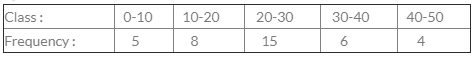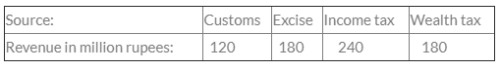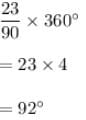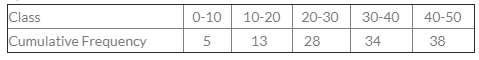# Test: Statistical Description Of Data - 3

## 40 Questions MCQ Test Quantitative Aptitude for CA CPT | Test: Statistical Description Of Data - 3

Description
Attempt Test: Statistical Description Of Data - 3 | 40 questions in 40 minutes | Mock test for CA Foundation preparation | Free important questions MCQ to study Quantitative Aptitude for CA CPT for CA Foundation Exam | Download free PDF with solutions
QUESTION: 1

Solution:
QUESTION: 2

Solution:
QUESTION: 3

### From which graphical representation, we can calculate partition values?

Solution:
QUESTION: 4​For the class 20-30, cumulative frequency is

Solution:
QUESTION: 5

Difference between the maximum and minimum value of a given data is called

Solution:
QUESTION: 6

The best method of presentation of data is

Solution:
QUESTION: 7

A pie diagram is used to represent the following data:The central angles in the pie diagram corresponding to income tax and wealth tax respectively:

Solution:
QUESTION: 8

The following data relates to the marks of a group of  students.
Marks                         No. of Students
More than 70%                          07
More than 60%                          18
More than 50%                          40
More than 40%                          60
More than 30%                          75
More than 20%                         100
How many students have got marks less than 50%?

Solution:

Explanation : 40 students got marks more than 50%

Let Total students = 100

Students got marks less than 50% =  100 - 40

= 60 students.

QUESTION: 9

If calss interval is 10-14, 15-19, 20-24, then the first class is

Solution:
QUESTION: 10

Which of the following is not a two dimensional diagram?

Solution:
QUESTION: 11

The difference between the upper and lower limit of a class is called ___________.

Solution:
QUESTION: 12

To draw Histogram, the frequency distribution should be:

Solution:
QUESTION: 13

Cost of Sugar in a month under the heads raw materials, labour, direct production and others were 12, 20, 35 & 23 units respectively. The difference between their central angles for the largest & smallest components of the cost of Sugar is

Solution:

Since we have given that
12, 20, 35, 23 units.
Total units would be
12 + 20+ 35 + 23 = 90 Units
Difference between largest and smallest components is given by
35 - 12 = 23
So, the central angle for the difference would beQUESTION: 14

What is a exclusive series?

Solution:
QUESTION: 15

The breadth of the rectangle is equal to the length of the class interval in

Solution:
QUESTION: 16

The frequency of class 20-30 in the following dataSolution:
QUESTION: 17

Frequency Density can be termed as:

Solution:
QUESTION: 18

The Graphical representation by which median is calculated is called

Solution:
QUESTION: 19

Arrange the following dimension wise : pie-diagram, bar-diagram and cubic diagram.

Solution:
QUESTION: 20

For the overlapping classes 0-10, 10-20, 20-30 etc. The class mark of the class 0-10 is

Solution:
QUESTION: 21

In Histogram, the classes are taken

Solution:
QUESTION: 22

The curve obtained by joining the points, whose x-coordinates are the upper limits of the class-intervals and y coordinates are corresponding cumulative frequencies is called

Solution:
QUESTION: 23

For non-overlapping classes 0-19, 20-39, 40-59 the class mark of the class 0-19 is

Solution:
QUESTION: 24

The Chronological classification of data are classified on the basis of:

Solution:
QUESTION: 25

An ogive can be prepared in ____________different ways.

Solution:
QUESTION: 26

Diagrammatic representation of the cumulative frequency distribution is

Solution:
QUESTION: 27

For overlapping class-intervals the class limit & class boundary are

Solution:
QUESTION: 28

Classification is of

Solution:

QUESTION: 29

Upper boundary of any class coincides with the lower boundary of the next class.

Solution:
QUESTION: 30

For obtaining frequency polygon we join the successive points whose abscissa represent the corresponding class frequency________

Solution:
QUESTION: 31

Excepting the first and the last, all other class boundaries lie midway between the upper limit of a class and the lower limit of the next higher class.

Solution:
QUESTION: 32

Difference between the maximum & minimum value of a given data is called

Solution:
QUESTION: 33

When one end of a class is not specified, the class is called

Solution:
QUESTION: 34

The lower extreme point of a class of called

Solution:
QUESTION: 35

When the width of all classes is same, frequency polygon has not the same area as the Histogram.

Solution:
QUESTION: 36

To find the mode graphically

Solution:
QUESTION: 37

When all classes have equal width, the heights of the rectangles in Histogram will be numerically equal to the

Solution:
QUESTION: 38

Histogram emphasizes the widths of rectangles between the class boundaries.

Solution:
QUESTION: 39

The difference between the upper boundary and the lower boundary of a class is called

Solution:

Class size is the difference between the upper boundary and the lower boundary of the class.

QUESTION: 40

Which of the following can be determined by using the Ogive curve?

Solution:

Both median and quartiles can be determined from the Ogive curve.Use Code STAYHOME200 and get INR 200 additional OFF Use Coupon Code